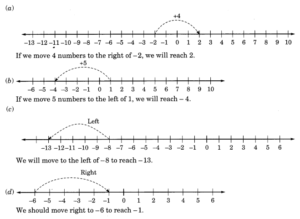# Ncert Class 6 Math Integers Exercise 6.1

Integers Class 6 Ex. 6.1

New Ncert Class 6 Math Free Solution.

Exercise 6.1

Question 1 :- Write down opposites of the following:
(a) Increase in weight
(b) 30 km North
(c) 326 BC
(d) Loss of ₹700
(e) 100 m above sea level.

Solution 1:-
(a) Decrease in weight
(b) 30 km South
(d) Profit of ₹700
(e)100 m below sea level.

Question 2 :- Represent  following numbers as integers with appropriate signs.

(a) An aeroplane is flying at a height-of two-thousand metre(m) above the ground.
(b) A submarine is moving at a depth of eight-hundred metre below the sea level.
(c) A deposit of rupees two hundred.
(d) Withdrawal of rupees seven hundred.

Solution 2:-
(a) +2000 m
(b) -800 m
(c) + ₹200
(d) – ₹700

Question 3 :- Represent the following numbers on a number line:

(a) +5
(b) -10
(c) +8
(d) -1
(e) -6

Solution 3:-
(a) +5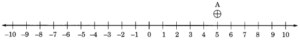(b) -10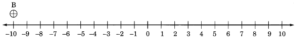(c) +8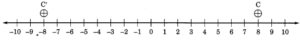(d) -1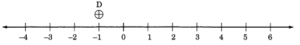(e) -6Question 4 :- The adjacent figure is a vertical number line, representing integers. Observe it and locate the following pointss: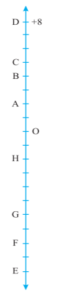(a) If point D is +8, then which point is -8?
(b) Is point G a negative-integer or a positive-integer ?
(c) Write integer for points B and E.
(d) Which point marked on this number line has, the least value?
(e) Arrange all the pointss in decreasing-order of values.

Solution 4:-
(a) F represents -8
(b) G is a negative integer.
(c) B represents +4 and E represents – 10
(d) The value of E is the lowest at -10.
(e) The points are listed in decreasing sequence as follows: D, C, B, A, O, H, G, F, and E.

Question 5 :- Following is the list of the temperatures of five places in India on a particular day of the year.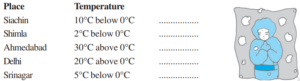(a) Write the temperaturess of these places in the form of integers in the blank-column ?
(b) Following is the number line representing the temperatures in degree-Celsius.
Plot the names of the city against its temperature.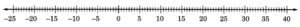(c) Which is the coolest place?
(d) Write the names of the places where temperatures, above the 10°C.

Solution 5:- (a)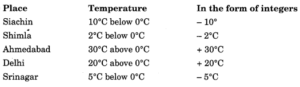(b)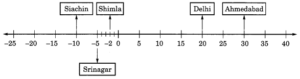(c) The coldest place is Siachin, where the temperature is -10°C.

(d) (1) Delhi → 20°C

Question 6 :- In each of the following pair, which of the number is to the right of the other on the number line?
(a) 2, 9
(b) -3, -8
(c) 0, -1
(d) -11, 10
(e) -6, 6
(f) 1, -100

Solution 6:-

(a) 9 is to the right of 2.
(b) -3 is to the right of -8
(c) Right of -1 is 0, and vice versa.
(d) 10 is to the right of -11.
(e) 6 is to the right of -6
(f) 1 is located to the right of -100.

Question 7 :- Write all the integer between the given pair (write them in the increasing order):
(a) 0 and -7
(b) -4 and 4
(c) -8 and -15
(d) -30 and -23

Solution 7:-

(a) Integers between 0 and -7 are:
– 6,  – 5,  – 4,  -3,  -2,  – 1.
(b) Integers between -4 and 4 are:
– 3,  – 2,  -1,  0,  1,  2,  3.
(c) Integers between -8 and -15 are:
-14,-13, -12,-11,-10,-9.
(d) Integers between -30 and -23 are:
– 29, – 28, – 27, – 26, – 25, – 24.

Question 8 :- (a) Write the four negative-integers greater than -20.
(b) Write the four negative-integers less than – 10.

Solution 8:-
(a) There are four negative integers greater than – 20 are: – 19, – 18, – 17, – 16.
(b) There are four negative integers less than – 10 are: – 11, – 12, – 13, – 14.

Question 9 :- For the following statement, write the True (T) or False (F). If the statement is false, so correct the statement.
(a) – 8; is to the right of  -10 on a number line.
(b) – 100; is to the right of  -50 on a number line.
(c) Smallest negative integer is – 1
(d) -26; is greater than -25.

Solution 9:-

(a) True (T)
(b) False (F);
(c) False (F);
(d) False (F); Correction: -26 is smaller than -25.

Question 10 :- Draw a number Line and answer the following:

(a) Which number will we reach; if we move 4 numbers to the right of -2.
(b) Which number will we reach; if we move 5 numbers to the left of 1.
(c) If we are at -8 on the number line, in which direction should we move, to reach -13 ?
(d) If we are at -6 on the number line, in which direction should we move, to reach -1 ?

Solution 10:-